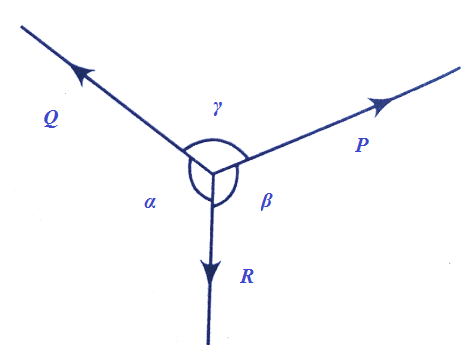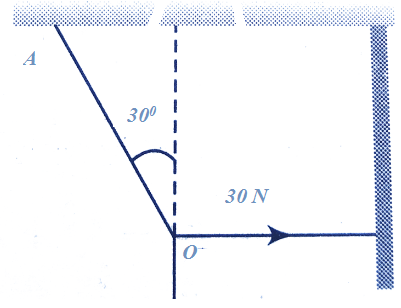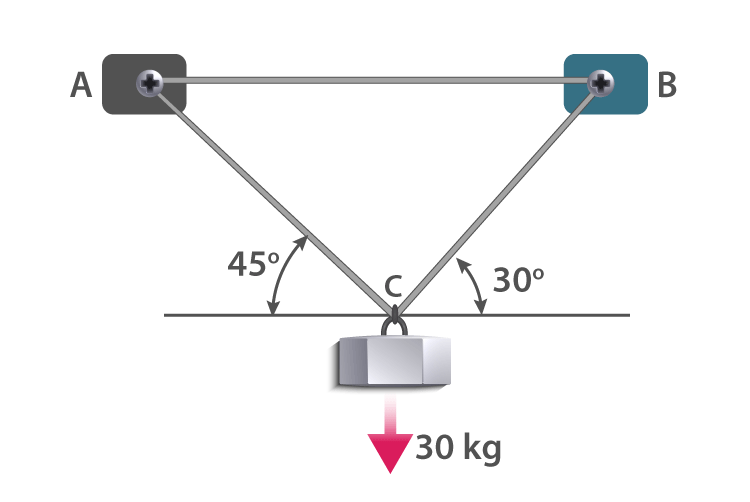# Lami’s Theorem MCQs for NEET

Lami’s theorem states that three forces are said to be in equilibrium when each force is proportional to the sine of the other two forces. Lami’s theorem relates the magnitudes of concurrent, coplanar and non-collinear forces that maintain an object in static equilibrium. The theorem is useful in analyzing mechanical and structural systems.

1. Three coplanar forces A, B and C acting at a point in the plane are in equilibrium. If the given value of A is 1.9318 kg wt and sinθ1 is 0.9659, what is the value of C?

1. 1
2. 2
3. 0.9659
4. ½

2. A body under the action of coplanar forces X, Y and Z, is in equilibrium as shown in the figure. Which of the following is the correct statement?1. $$\frac{P}{\sin \alpha }=\frac{Q}{\sin \beta}=\frac{R}{\sin \gamma}$$
2. $$\frac{P}{\cos \alpha }=\frac{Q}{\cos \beta}=\frac{R}{\cos \gamma}$$
3. $$\frac{P}{\sin \alpha }=\frac{Q}{\cos \beta}=\frac{R}{\tan \gamma}$$
4. $$\frac{P}{\tan \alpha }=\frac{Q}{\tan \beta}=\frac{R}{\tan \gamma}$$

Answer: (a) $$\frac{P}{\sin \alpha }=\frac{Q}{\sin \beta}=\frac{R}{\sin \gamma}$$

3. If the body is under equilibrium under the influence of a set of non-colinear force, then the minimum number of forces has to be

1. Two
2. Three
3. Four
4. Five

4. What is the minimum number of non-zero vectors in different planes that can be added to give a resultant of zero?

1. Two
2. Three
3. Four
4. Five

5. In the given figure, the tension in the horizontal cord is 30 N. What is the weight W and tension in the string OA in Netwon?1. $$30\sqrt{3}$$, 30
2. $$60\sqrt{3}$$, 30
3. $$30\sqrt{3}$$, 60
4. $$60\sqrt{3}$$, 60

Answer: (c) $$30\sqrt{3}$$, 60

6. According to Lami’s theorem which of the following statements is true?

1. Three forces acting at a point will be in equilibrium.
2. Three forces acting upon a particle will be in equilibrium if they are represented in magnitude and direction by the sides of a triangle, taken in order.
3. If three forces acting at a point are in equilibrium, each force is proportional to the sine of the angle between the other two.
4. Three forces acting at a point can be represented by a triangle, each side being proportional to the force.

Answer: (c) If three forces acting at a point are in equilibrium, each force is proportional to the sine of the angle between the other two.

7. Which of the following theories states that “if a body is in equilibrium under the action of three coplanar and concurrent forces, each of the forces is proportional to the sine of the angle between the other two.”

1. Parallelogram Law of Forces
2. Lami’s Theorem
3. Triangle Law of Forces
4. Polygon Law of Forces

8. Which of the following is true about Lami’s theorem?

1. The theorem is derived based on the cosine rule of trigonometry
2. The theorem is only applicable to regular shaped bodies in equilibrium
3. The theorem is helpful in determining the unknown forces acting at a point for an object in equilibrium.
4. The theorem determines the forces acting on a moving body.

Answer: (c) The theorem is helpful in determining the unknown forces acting at a point for an object in equilibrium.

9. A 30 kg iron block is suspended using supports A and B as shown in the figure. What is the tension in both ropes?1. 263.566 N and 215.2 N
2. 463.566 N and 415.2 N
3. 663.566 N and 615.2 N
4. 863.566 N and 815.2 N

Answer: (a) 263.566 N and 215.2 N

10. In the figure given below, the advertisement board having a mass of 6 kg is suspended by two strings which makes an angle of 450 with the signboard. How much is the tension in both the strings?1. 21.6 N
2. 41.6 N
3. 61.6 N
4. 81.6 N